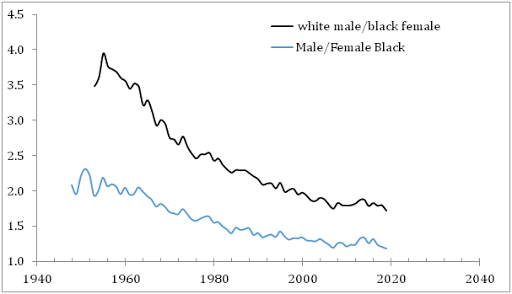## 2/7/21

### It is highly likely that real economic growth in the USA in overestimated by 26% since 1979

We discussed the problem of inflation definition in the USA in many posts (e.g., this one). The principal finding is that the CPI and GDP deflator, dGDP, started to deviate in 1979 due to the introduction of new price inflation definition. Figure 1 presents the CPI and dGDP and gives a clear understanding that the dGDP is just the CPI divided by 1.22. This is an important observation for an unbiased real GDP per capita estimate in the USA. The new definition is highly likely was introduced to obtain a larger real GDP per capita than was calculated with the CPI. Figure 2 presents the real GDP per capita reported by the BEA and that recalculated using the ratio of the CPI and dGDP. One can see that the GDP per capita in 2019 should be \$39,500 instead of the reported value of \$49,649, i.e. only 80% of the current level.

It is highly likely that real economic growth in the US in overestimated by 26% since 1979

Figure 1. The CPI and dGDP in the USA. After 1979, dGDP=CPI/1.22.

Figure 2. The GDP per capita estimates in the USA as published by the BEA and that corrected with the difference between the dGDP and CPI.

### The mean income gap between white males and black females grows during the democratic presidencies

Two days ago, we compared the mean income evolution of the white and black population and demonstrated that the difference did not change mu...